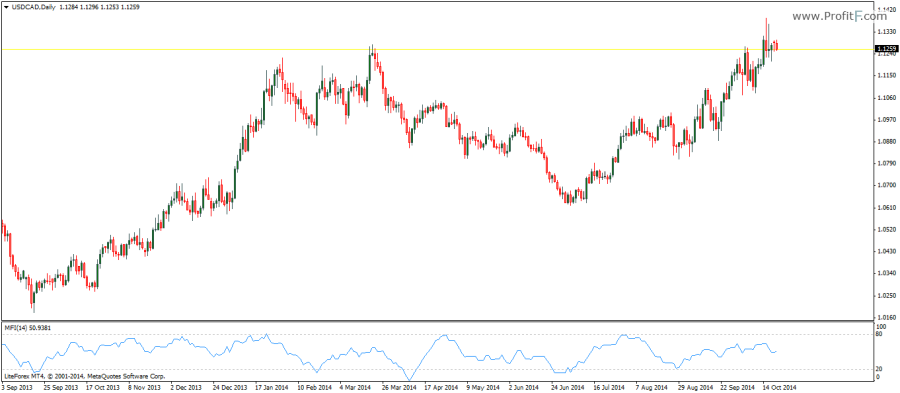# Money Flow index Indicator

Money Flow index Indicatoror MFI for short is an indicator measuring the rate of change at which money flows in and out of the security. The MFI indicator is closely related to the Relative Strength Index (RSI) with the main differentiating factor being that the volume is taken into consideration.

The MFI indicator is an oscillator which ranges between fixed values of 0 and 100 and as with most oscillators divergences form a major part of trading with the MFI indicator. The MFI indicator’s settings can be tweaked with the default parameters set to 14.The money flow index comprises various calculations with the first variable being the Typical Price, which is the average price of the High/Low and Close. The Typical Price is then multiplied by its corresponding volume. Volume is calculated as positive if the volume for current day is more than the volume for the previous day known as Positive Money Flow and when the current volume is lesser than the previous volume, it is known as Negative Money Flow. The final calculation of the MFI is derived from the above values. Because the oscillator ranges between 0 and 100, the MFI can also be used to determine overbought and oversold levels in ranging markets.

Rate this post:(No Ratings Yet)Loading...
---